Swift Talk # 223

## Drawing Trees (Part 1)

### This episode is freely available thanks to the support of our subscribers

We experiment with different tree-drawing algorithms to produce readable and compact binary trees.

00:06 Today we're going to draw binary trees. We want to feature tree diagrams in our slides framework, but what we build today can also be used independently.

00:26 For the illustrations in our Thinking in SwiftUI book, we built tree layouts using `VStack`s and `HStack`s. However, this produced trees with suboptimal space use, because subtrees would never overlap in any way, leaving more spaces empty than necessary. It worked fine for what we needed in the book, but more complex trees can be laid out in a more optimal way.

01:19 There's a great article that discusses drawing neat trees in Python: Drawing Presentable Trees by Bill Mill. We'll use some of the principles and algorithms described in there.

01:35 We've prepared most of the stuff we need, so we don't have to waste any time on the actual drawing of the nodes and the connecting lines. Each node is presented as a circle with buttons for adding left and right subtrees. Only the layout logic is still missing, so when we add a child node, it currently gets drawn over the parent node: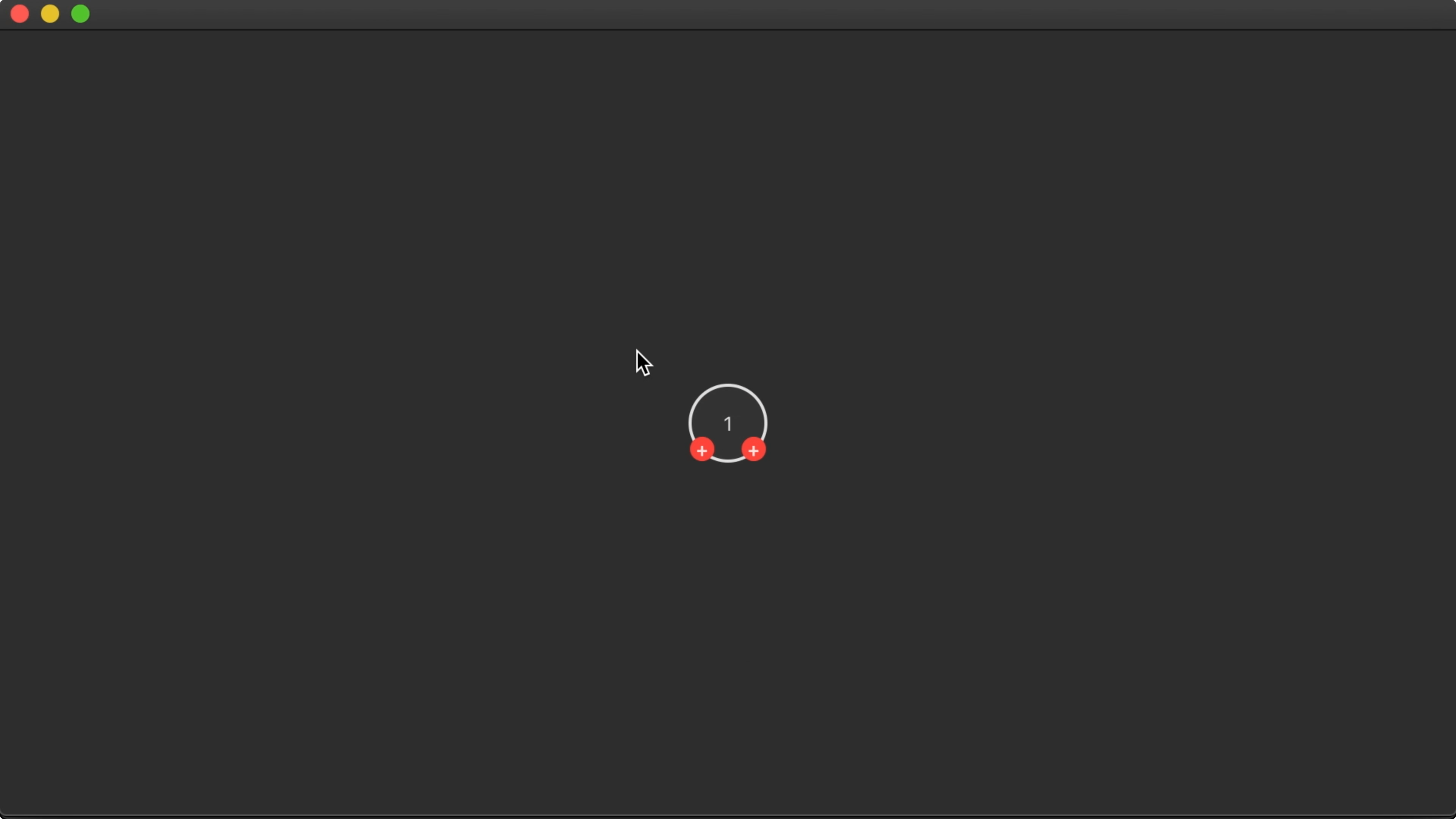## Tree Class

02:09 The data structure with which we build up the tree is a class, `Tree`. It holds a `value` and optional `left` and `right` subtrees. It also has a `point` property that contains two integer coordinates, `x` and `y`:

``````struct Point: Hashable {
var x: Int
var y: Int

static let zero = Point(x: 0, y: 0)
}

final class Tree<A>: ObservableObject, Identifiable, CustomStringConvertible {
// ...
@Published var value: A
@Published var point: Point = .zero
@Published var left: Tree<A>? {
didSet { left?.parent = self }
}
@Published var right: Tree<A>? {
didSet { right?.parent = self }
}
weak var parent: Tree<A>? = nil
// ...
}
``````

02:40 The `point` value defines a node's position in the tree. We lay out all nodes according to these values, and then we draw edges between each node and its parent.

03:00 Most of the time in SwiftUI, we model data using structs and enums, but a reference type makes demoing today's algorithms much easier, so that's why we chose to implement `Tree` as a class.

03:38 The first algorithm we'll implement is a straightforward one by Donald Knuth. Starting with the root node, it lays out the left subtree, then the node itself, and then the right subtree. Each node's `x` coordinate comes from a running counter, and its `y` coordinate is equal to its depth in the tree.

## Layout

04:36 But before we implement the algorithm, we have to implement the `relayout` method stub. This method gets called on the node whose subtrees have changed. If this happens, we have to lay out the entire tree again. So we traverse up to the root node and we call `layout` on it:

``````final class Tree<A>: ObservableObject, Identifiable, CustomStringConvertible {
// ...
func relayout() {
var root = self
while let p = root.parent {
root = p
}
root.layout()
}
}
``````

## Knuth

05:31 In an extension on `Tree`, we can implement the algorithm itself in the `layout` method. We need a running `x` coordinate, which we model as an in-out parameter. And we add another parameter for passing in the depth of the node we're laying out:

``````extension Tree {
func layout() {
var x = 0
knuth(depth: 0, x: &x)
}

func knuth(depth: Int, x: inout Int) {

}
}
``````

06:35 We first lay out the left subtree recursively. Then we assign the current node's coordinates, incrementing the running `x` by `1`. Finally, we lay out the right subtree:

``````extension Tree {
func layout() {
var x: [Int:Int] = [:]
alt(depth: 0, x: &x)
}

func knuth(depth: Int, x: inout Int) {
left?.knuth(depth: depth + 1, x: &x)
point.x = x
point.y = depth
x += 1
right?.knuth(depth: depth + 1, x: &x)
}
``````

07:44 This works well for simple trees. But because there will never be nodes that share an `x` coordinate, the algorithm can result in suboptimal layouts, depending on the tree structure: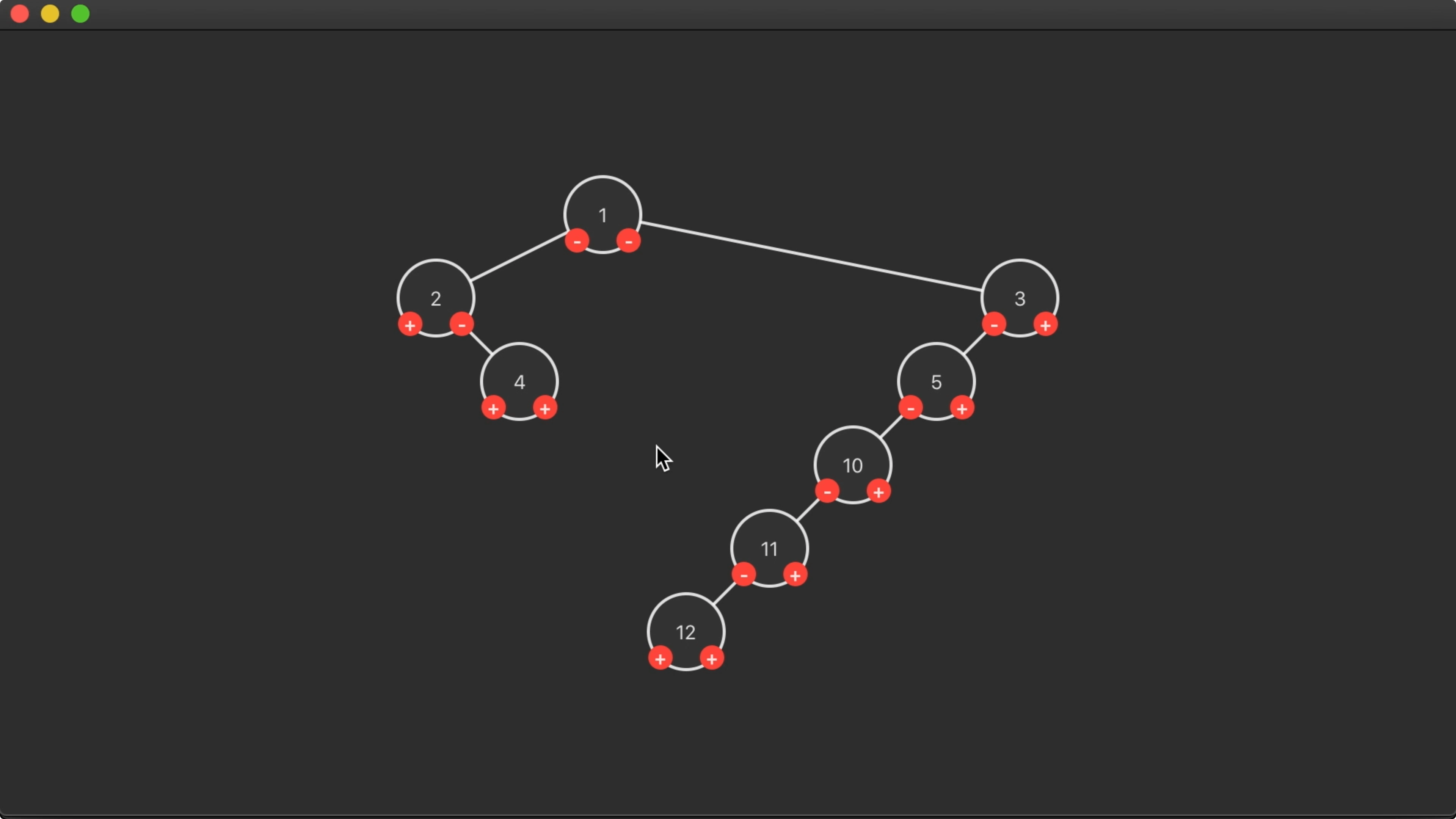In this case, it would be fine for the right subtree to move to the left, with some nodes below each other sharing the same `x` coordinate.

## Alternative

09:02 We can improve the algorithm by keeping a running `x` for every depth. So instead of a single `x` value, we use a dictionary that maps depths to available `x` positions. When setting a node's `point`, we use the `x` entry for the node's depth — defaulting to zero — and then we increment the available position for that depth by `1`:

``````extension Tree {
func layout() {
var x: [Int:Int] = [:]
alt(depth: 0, x: &x)
}

func alt(depth: Int, x: inout [Int:Int]) {
left?.alt(depth: depth+1, x: &x)
point.x = x[depth, default: 0]
point.y = depth
x[depth, default: 0] += 1
right?.alt(depth: depth+1, x: &x)
}
}
``````

10:59 This produces extremely compact trees, but the layout actually makes it hard to read the tree: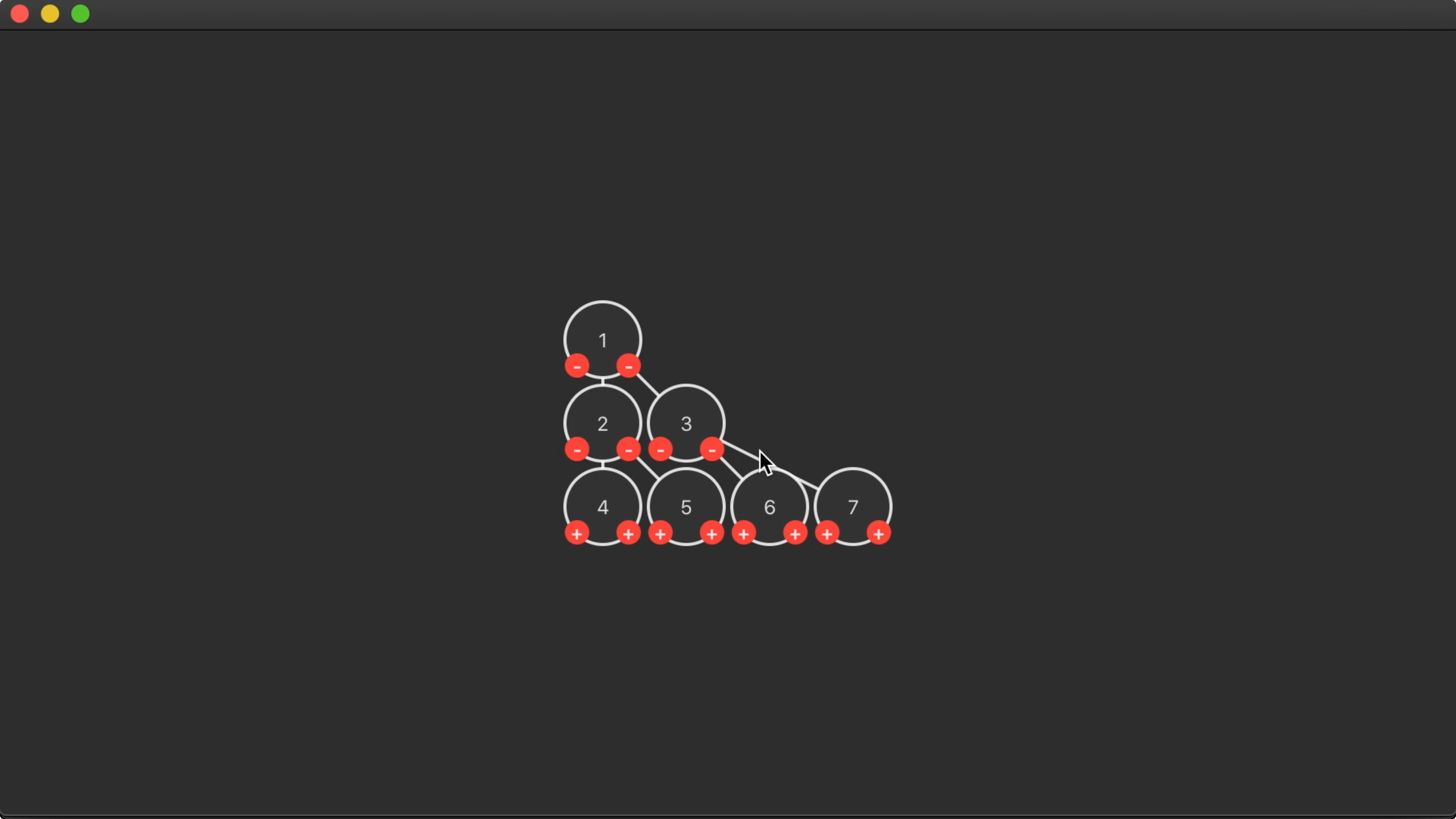11:55 Both the article we're basing this work on and the papers it cites offer a few principles for drawing aesthetically pleasing trees. We're already adhering to some principles — e.g. nodes at the same depths are drawn on the same horizontal line — but we should adopt another important one: each parent node is centered above its children.

12:34 We went from a readable but space-wasting tree to a very compact tree that's hard to read. We can try to get some readability back by adding more space where needed. For one, we should move a node to the right if it has a left subtree:

``````extension Tree {
func layout() {
var x: [Int:Int] = [:]
alt(depth: 0, x: &x)
}

func alt(depth: Int, x: inout [Int:Int]) {
left?.alt(depth: depth+1, x: &x)
point.x = x[depth, default: 0]
if let _ = left {
point.x += 1
}
// ...
}
``````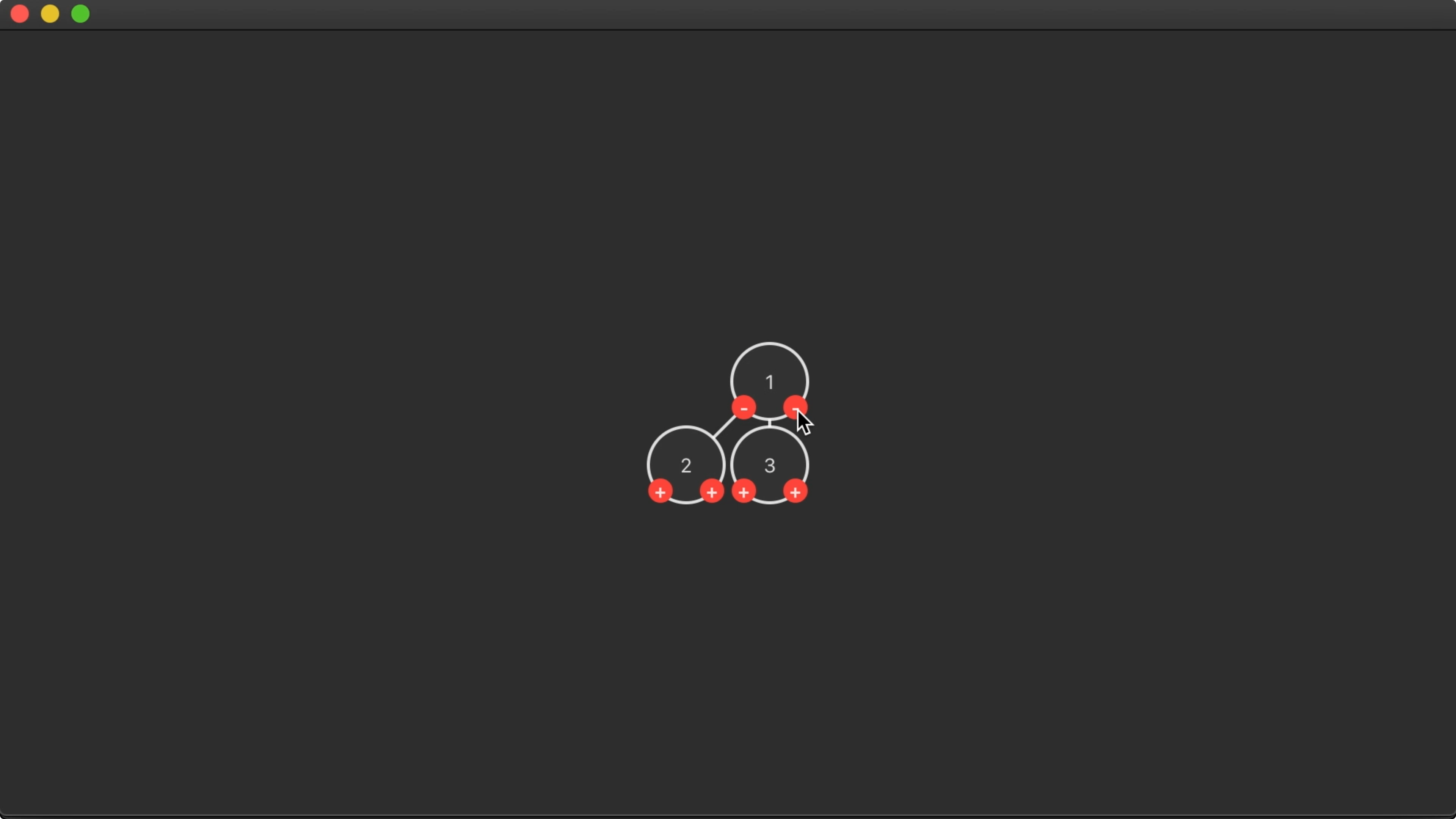14:28 And to balance the tree, we also have to move the right subtree to the right by one position. We do so, after laying out the right subtree, by using the `modifyAll` method on `Tree` to transform every node:

``````extension Tree {
func layout() {
var x: [Int:Int] = [:]
alt(depth: 0, x: &x)
}

func alt(depth: Int, x: inout [Int:Int]) {
left?.alt(depth: depth+1, x: &x)
point.x = x[depth, default: 0]
if let _ = left {
point.x += 1
}
point.y = depth
x[depth, default: 0] = point.x + 1
right?.alt(depth: depth+1, x: &x)
right?.moveRight(1)
}

func moveRight(_ amount: Int) {
modifyAll { \$0.point.x += amount }
}
}
``````

15:52 This works great for a simple tree: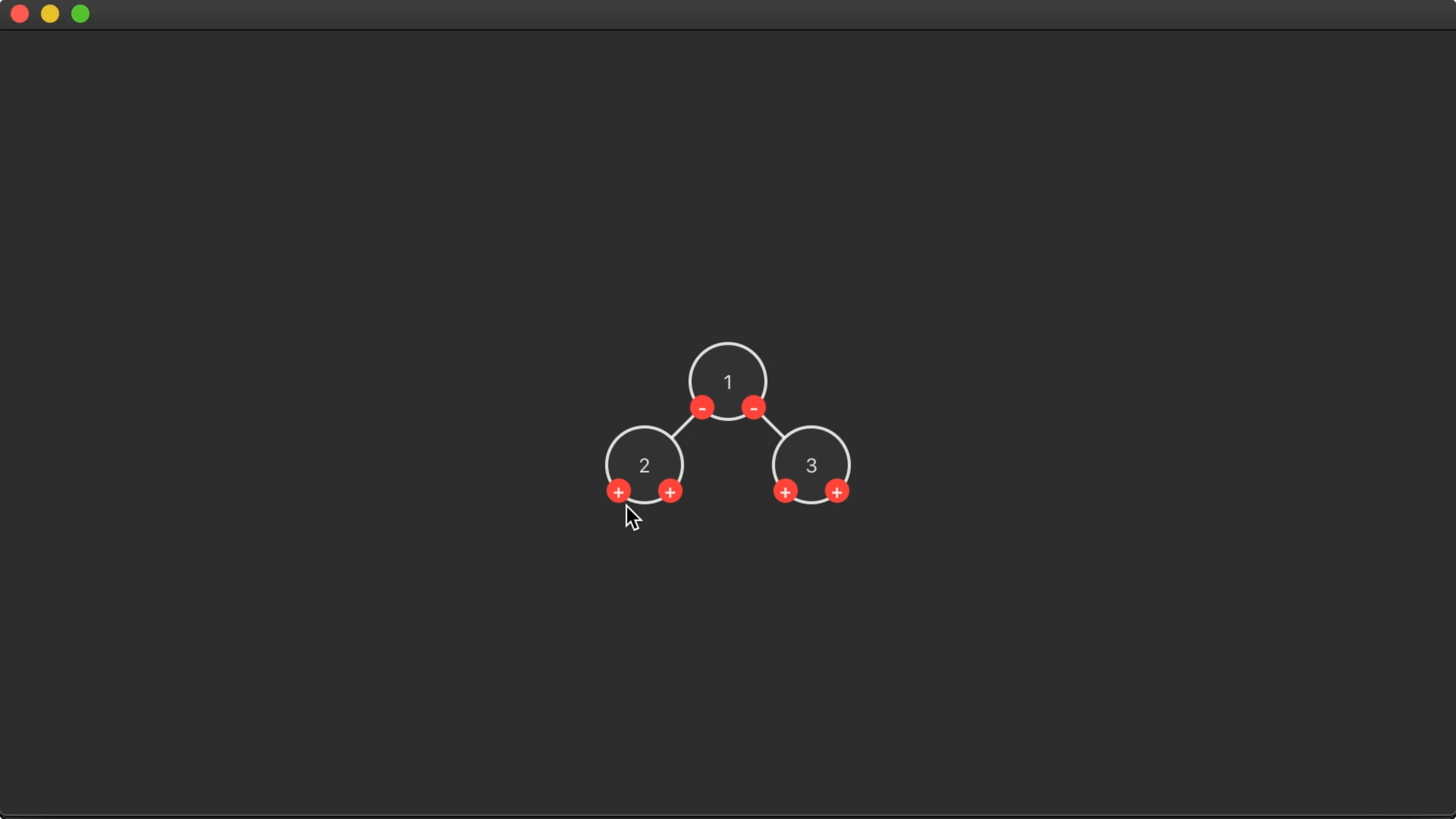16:05 But things get weird when we add more nodes. Here we can see that the tree is left-biased and nodes can overlap each other: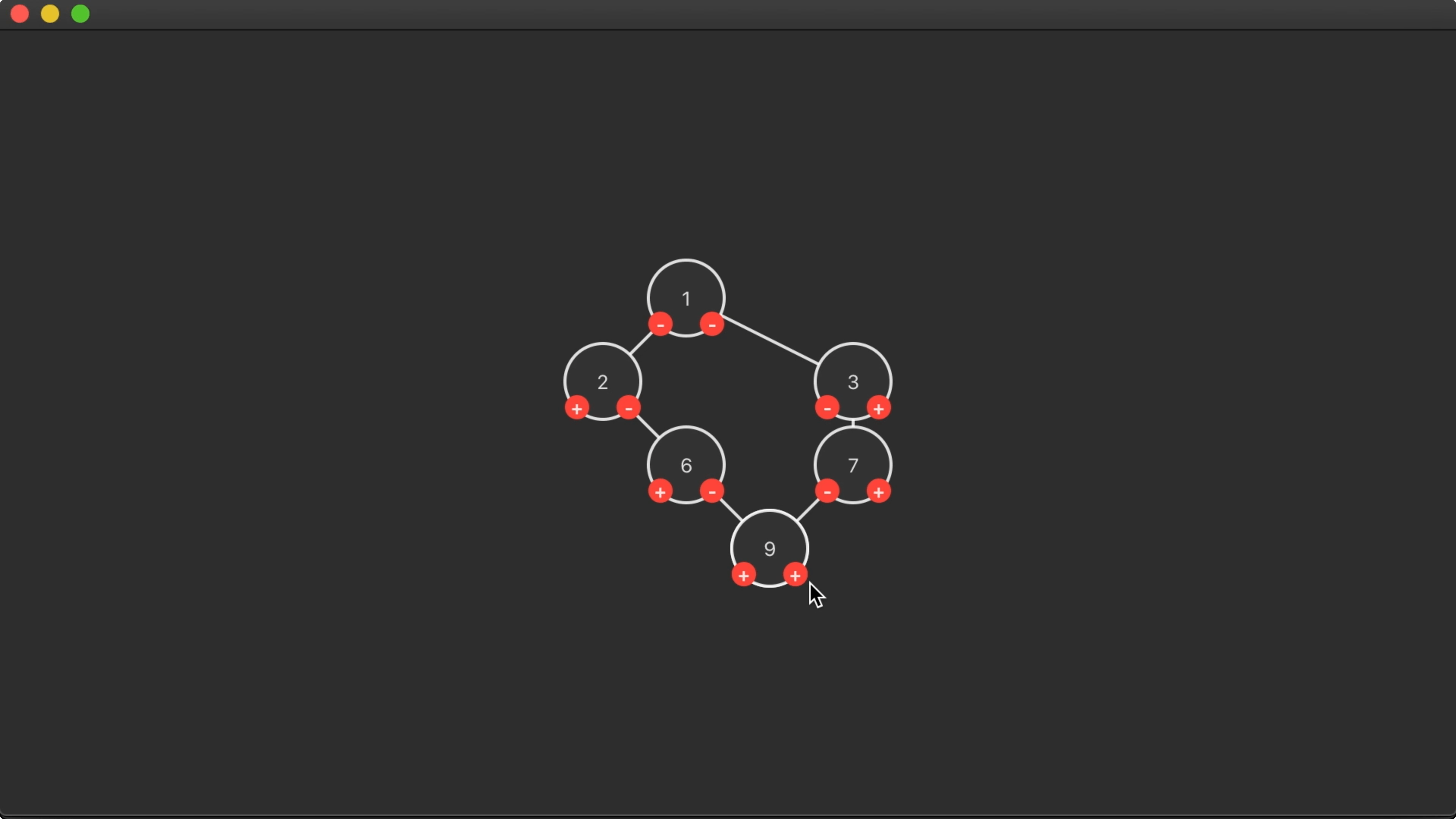16:33 As we move the right subtree to the right, we should adjust the dictionary with available `x` positions accordingly:

``````extension Tree {
// ..
func alt(depth: Int, x: inout [Int:Int]) {
// ...
right?.moveRight(1)
for (d, v) in x {
if d > depth {
x[d] = v + 1
}
}
}
// ...
}
``````

17:44 However, we might be updating some `x` entries unnecessarily, causing nodes to be placed too far to the right. In some cases, this makes it hard to see whether a subtree is to the left or right of its parent node: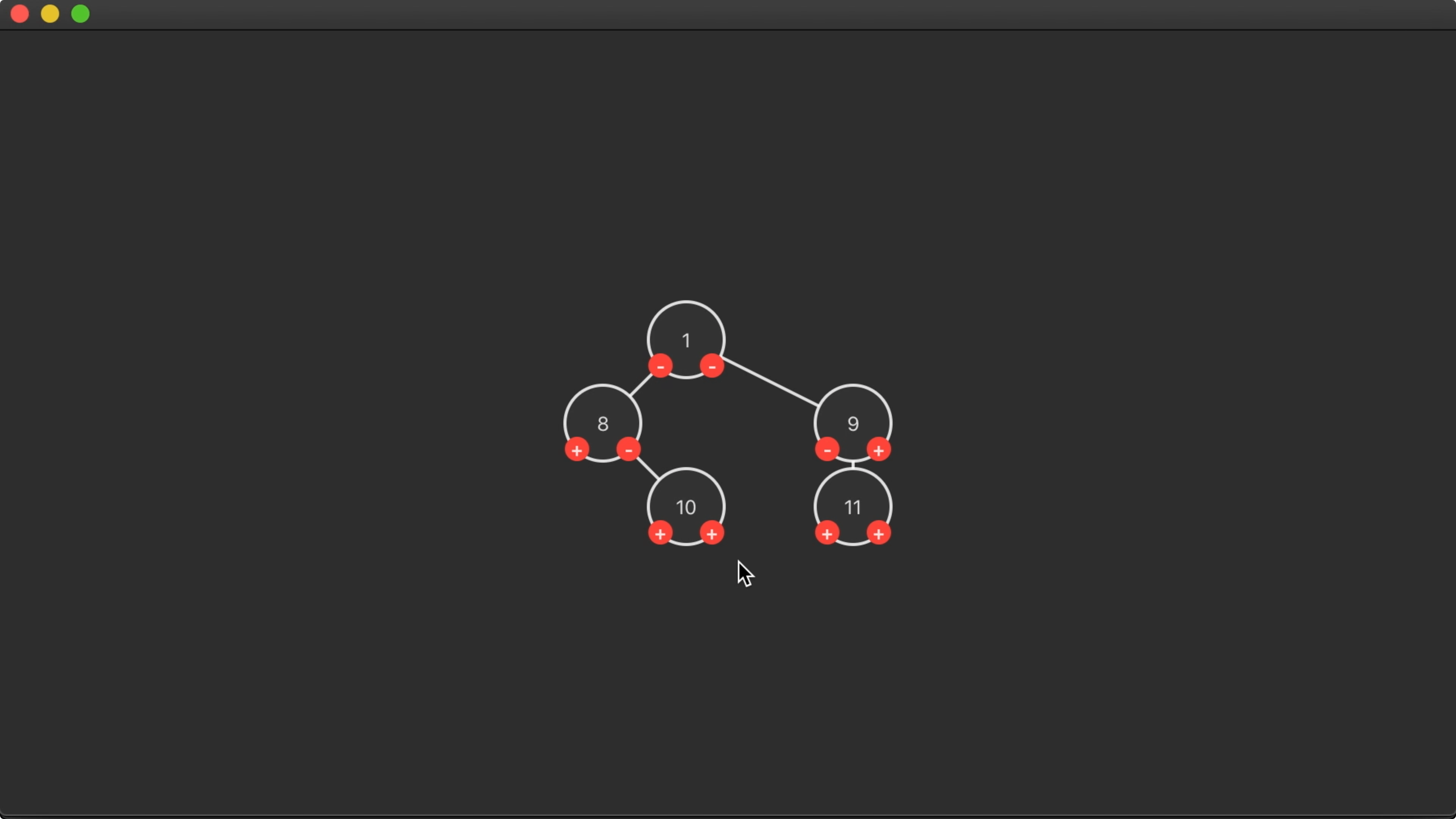18:23 We can partially fix this by making sure each node is positioned to the right of its left subtree:

``````extension Tree {
// ..
func alt(depth: Int, x: inout [Int:Int]) {
left?.alt(depth: depth+1, x: &x)
point.x = x[depth, default: 0]
if let l = left {
point.x = l.point.x + 1
}
point.y = depth
x[depth, default: 0] = point.x + 1
// ...
}
// ...
}
``````

18:48 That fixes the configuration of parents and children, but we're still seeing some gaps that could be smaller. Also, some nodes aren't centered correctly above their children: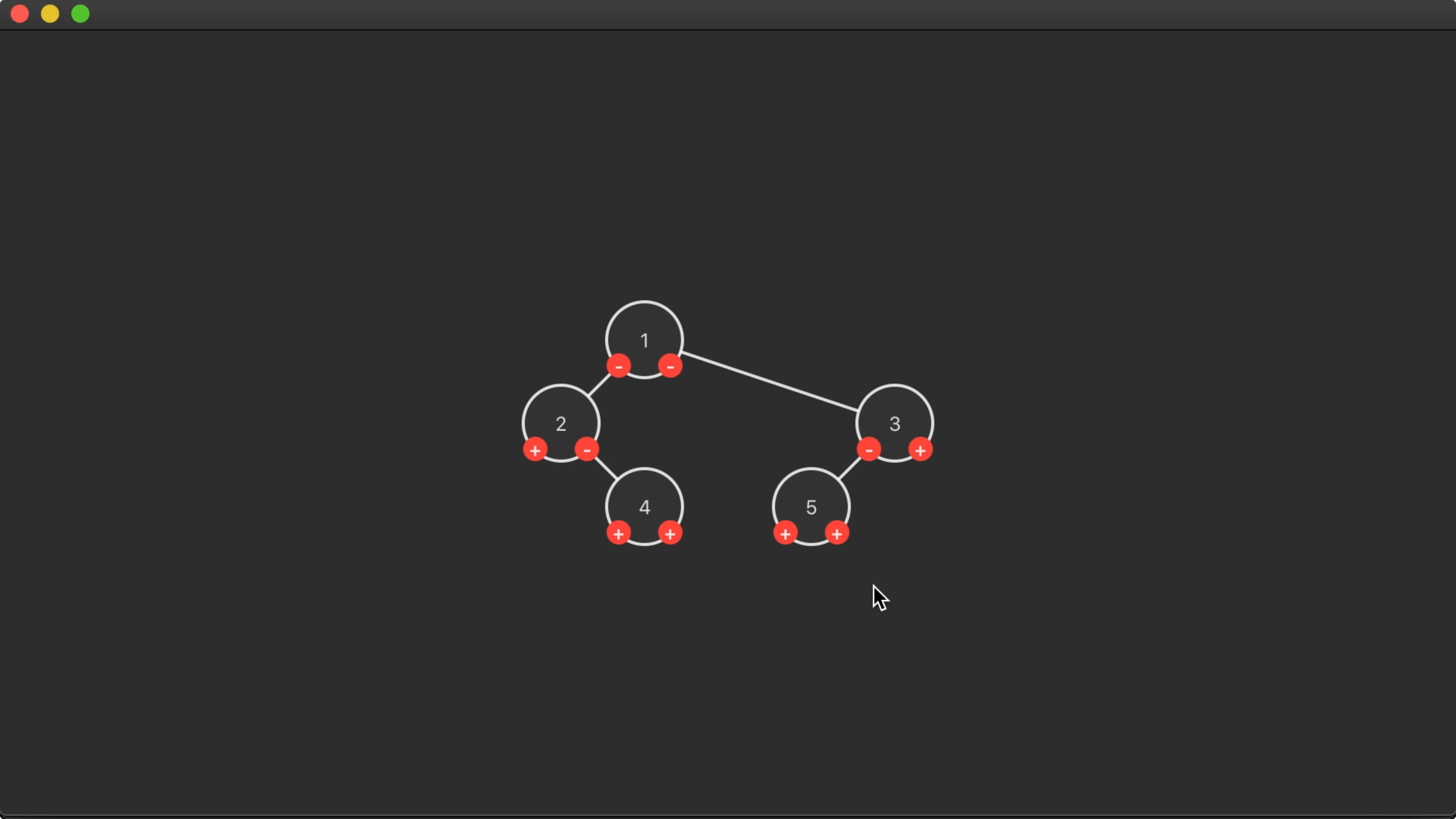## Next Time

19:28 The overall result is a slightly more compact yet readable tree. But it isn't perfect. The tree is left biased, which makes the right subtrees look a little funny. And we're not sure if we can efficiently improve this algorithm to yield better results.

20:31 In the next episode, we'll take a different approach and build the tree from the bottom up. By laying out the left and right subtrees and then figuring out how to position the subtrees so that they don't overlap, we can hopefully draw a more symmetrical tree.

### Resources

• #### Sample Code

Written in Swift 5.3

See All
Experiments

### Git Under the Hood: Blobs

Episode 357 · May 26

SwiftUI

### Connecting Lines with Anchors (Part 3)

Episode 356 · May 19

SwiftUI

### Connecting Lines with Anchors (Part 2)

Episode 355 · May 12

SwiftUI

### Connecting Lines with Anchors (Part 1)

Episode 354 · May 05

SwiftUI

### Reimplementing Anchors: Transforms

Episode 353 · Apr 28

SwiftUI

### Reimplementing Anchors: Points

Episode 352 · Apr 21

SwiftUI

### Reimplementing Anchors: Bounds

Episode 351 · Apr 14

SwiftUI

### Flow Layout Alignment

Episode 350 · Apr 07

Unlock Full Access

## Subscribe to Swift Talk

• ### Watch All Episodes

A new episode every week